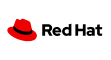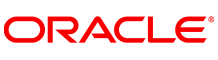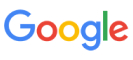New update is available. Click here to update.
Topics

# Jumping Numbers

Hard0/120
Average time to solve is 10m+2 more companies

## Problem statement

You are given a positive integer 'N'. Your task is to print all the jumping numbers smaller than or equal to 'N'.

A number is called a jumping number if all adjacent digits in it differ by 1. All the single-digit numbers are considered jumping numbers.

Note:

``````The difference between ‘9’ and ‘0’ is not considered as 1.
``````

Example:

``````Let’s say 'N' = 25. The jumping numbers less than or equal to 25 are: 0, 1, 2, 3, 4, 5, 6, 7, 8, 9, 10, 12, 21, 23. In all these numbers the adjacent digits differ by 1.
``````
Detailed explanation ( Input/output format, Notes, Images )
Constraints:
``````1 <= T <= 100
1 <= N <= 10^8

Time Limit: 1 sec
``````
Sample Input 1:
``````2
25
45
``````
Sample Output 1:
``````0 1 2 3 4 5 6 7 8 9 10 12 21 23
0 1 2 3 4 5 6 7 8 9 10 12 21 23 32 34 43 45
``````
Explanation 1:
``````For the first test case, refer to the example explained before.

For the second test case, N = 45. The jumping numbers less than 25 are: 0, 1, 2, 3, 4, 5, 6, 7, 8, 9, 10, 12, 21, 23, 32, 34, 43, 45.
``````
Sample Input 2:
``````2
10
1
``````
Sample Output 2:
``````0 1 2 3 4 5 6 7 8 9 10
0 1
``````Console